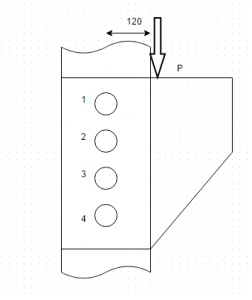Question

# Machine Layout

When two Belleville springs are in parallel, half force is obtained for a given deflection.
a) Half force
b) Double force
c) Same force
d) Can’t be determined

Explanation: Double force is obtained.

#### Earn Coins

Coins can be redeemed for fabulous gifts.

Similar Homework Help Questions
• ### Machine Layout

When two Belleville sprigs are arranged in series, half deflection is obtained for same force.a) One fourth deflectionb) Double deflectionc) Four time deflectiond) None of the listed

• ### Machine LayoutAre the bolts 2 and 3 under same force?a) Yesb) Noc) Depends on nature of force whether it is of shear or bending in natured) Can’t be determined

• ### Machine Layout

Belleville spring can only produce linear load deflection characteristics.a) Only linearb) Linear as well as non linearc) Non-lineard) None of the mentioned

• ### Machine Layout

For two springs connected in series, the net deflection is equal to the sum of deflection in two springs.a) Trueb) False

• ### Machine Layout

For two springs connected in parallel, net force is equal to the sum of force in each spring.a) Trueb) False

• ### Machine Layout

In drum brakes, as the temperature increases coefficient of friction ______a) increasesb) decreasesc) remains samed) Can’t be determined

• ### Machine Layout

Two plates each of thickness t are to be riveted together. If length of shank portion necessary to form the closing head is a, then length of rivet shank is given by?a) 2t+ab) 2(t+a)c) t+2ad) Can’t be determined

• ### Machine Layout

Can concentric springs be used to obtain a force which is not proportional to its deflection?a) Trueb) False

• ### Machine Layout

If the spring have same solid length and number of coils in the two springs are 8 and 10, then find the diameter of the spring with 8 coils. It is given diameter of spring with 10 coils is 12mm.a) 9.6mmb) 9mmc) 12mmd) 15mm

• ### Machine Layout

A railway wagon moving with a speed of 1.5m/s is brought to rest by bumper consisting of two springs. Mass of wagon is 100kg. The springs are compressed by 125mm. Calculate the maximum force acting on each spring.a) 1200Nb) 1500Nc) 1800Nd) 2000N